# 一文看懂深度学习手写体数字识别(MNIST)，小白学习总结

【摘要】 深度学习原理简单介绍 mnist手写体原理介绍

https://support.huaweicloud.com/bestpractice-modelarts/modelarts_10_0009.html

## 那么如何进行模型训练呢？先从最简单的数学原理开始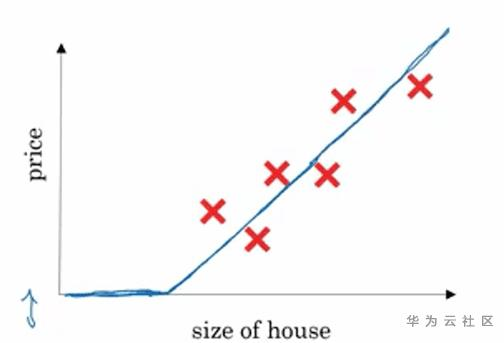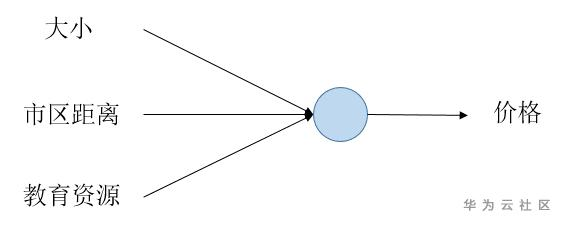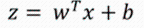Cost= Lost(y(1)) + Lost(y(2)) + ... + Lost(y(n))

## 二分分类Logistics的应用z是对应价格，而价格是个没有边界的值，可以从0到10万。但，如果我们要判断一个图像是不是猫，只能是一个概率值，就是这个图片是猫的概率是多少？概率是在（0,1）之间的。因此，我们需要做一个转换，把这个值转化成一个概率值。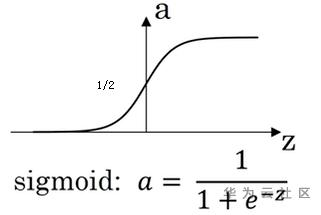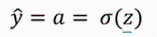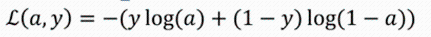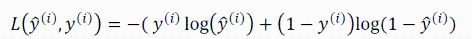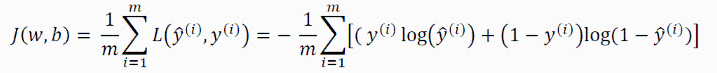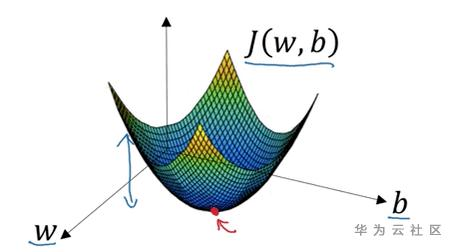......

## 回到我们的手写体识别，看看具体是怎么实现的

1、首先是数据的格式化。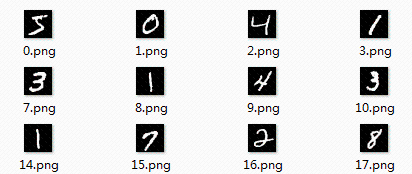y-hat = 1 * 10

W = 784 * 10

b = 1 * 10

x = 1 * 784

2、使用TensorFlow进行模型训练（使用开头说的ModelArts指导时则可以跳过这些代码步骤！）

TensorFlow是一个深度学习的框架，站在巨人的肩膀上，能够做的更快。也可以自己处理，那么就需要进行详细的代码编写，需要引用python的numpy包，用来高效计算矩阵。由于自己写的代码对CPU的调度能力不足，算法复杂度高，循环次数多等问题，训练模型时间异常久，能力不足只能靠框架了，使用TensorFlow训练数据，只要30秒就可以完成数据读取以及训练。

```mnist = inputdata.read_data_sets(=)

W = tf.Variable(tf.zeros([]))    b = tf.Variable(tf.zeros([])) x = tf.placeholder([])    y = tf.nn.softmax(tf.matmul(xW) + b)  y_ = tf.placeholder([])    cross_entropy = -tf.reduce_sum(y_ * tf.log(y))  train_step = tf.train.GradientDescentOptimizer().minimize(cross_entropy)```

`feed_dict={x: mnist.test.images, y_: mnist.test.labels}`

y函数的计算，这里用了另外一个激活函数sotfmax，和sigmoid函数一样，是把数值转化成概率的函数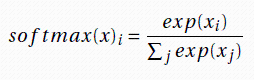cross_entropy就是我们说的cost函数，它统计了所有差异值，当然这里用的lost函数和我们之前的不一致

train_step这是一个声明使用梯度下降法训练模型的表达式，需要两个变量，一个是步长0.01，表示每次调整W,b需要调整多少量。另外一个是cost函数。表示期望要让哪个值达到最小。

```# 初始化变量，TensorFlow引擎需要的初始化
init = tf.global_variables_initializer()

sess = tf.Session() #初始化引擎
sess.run(init)  #初始化变量```

```# 开始循环模型， 循环1000次梯度下降的过程
for i in range(1000):
batch_xs, batch_ys = mnist.train.next_batch(100)  # mnist是数据集的所有数据
sess.run(train_step, feed_dict={x: batch_xs, y_: batch_ys})
# 每训练100次后评估模型状态
if i % 100 == 0:
correct_prediction = tf.equal(tf.argmax(y, 1), tf.arg_max(y_, 1))
accuracy = tf.reduce_mean(tf.cast(correct_prediction, "float"))

print(sess.run(accuracy, feed_dict={x: mnist.test.images, y_: mnist.test.labels}))

y_test = tf.nn.softmax(tf.matmul(input_array, W) + b)```

sess.run表示开启会话，TensorFlow需要使用会话的模式进行操作。

correct_prediction则表示，用预测值y去对比实际值y_，得到一个boolean型结果，如果匹配成功，则为true

## 最后总结

1、TensorFlow的使用，及框架学习

2、怎么继续提高手写体的识别率？

3、里面涉及到的各种细节操作。如lost函数的选取，激活函数的选取

1、首先是激活函数的选择

sigmoid、softmax等函数都是比较适用于概率模型的，能把数值转化成(0,1)的概率数值。当然有许多问题不是概率型的，因此这类函数就不一定适用，还有一些其他的函数，如ReLU，修正线性单元函数，ReLU=max(0,z)，比较常用的激活函数，还有tanh，sigmod函数向下平移0.5的变型函数，还有

leaky ReLU = max(0.01z,z)。激活函数的选择会影响学习过程中的一些权重变化。里面有很深的学问。

2、神经网络层数的选择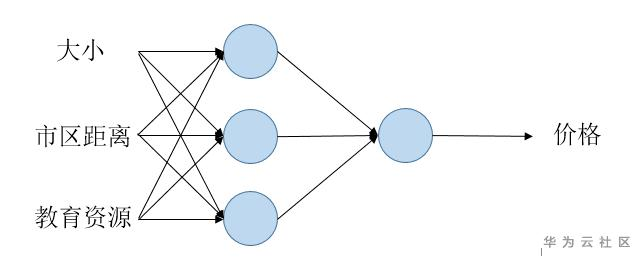3、神经网络神经元数量的选择

4、损失函数的选择

5、W和b初始值的选择

6、步长的选择

7、训练方法的选择

【版权声明】本文为华为云社区用户原创内容，转载时必须标注文章的来源（华为云社区），文章链接，文章作者等基本信息，否则作者和本社区有权追究责任。如果您发现本社区中有涉嫌抄袭的内容，欢迎发送邮件至：huaweicloud.bbs@huawei.com进行举报，并提供相关证据，一经查实，本社区将立刻删除涉嫌侵权内容。

### 评论 (0)

###### 温馨提示